# Bordering method

A method for solving a system of linear algebraic equations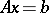with a non-degenerate matrix by inverting a matrix and calculating a determinant, based on passing recursively from the solution of the problem with matrixto the solution of the problem with the matrix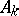, which can be considered as the result of bordering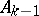.

The calculating scheme of the bordering method for the inversion of a matrix is as follows: Letbe a non-degenerate matrix. In the inversion of the matrix, the representation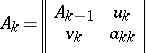(1)

is used, whereand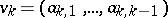. Then(2)Subsequent inversion of the matricesby this scheme gives the matrix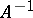.

The above scheme of the bordering method is only applicable to matrices with principal minors unequal to zero. Generally, a choice of the principal element is adopted. In this scheme, the bordering rows and columns used are those for which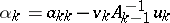has the maximum absolute value. The calculated matrix will then differ fromonly in the rearrangement of the rows and columns .

The bordering method is not the fastest of the direct methods of inversion of a matrix.

The bordering method permits an effective inversion of a triangular matrix. Ifis a right (upper-) triangular matrix, then in (1)The amount of calculation in this instance is reduced six-fold.

The bordering method is particularly effective when inverting Hermitian positive-definite matrices. For these matrices it is not necessary to use a scheme with a choice of a principal element. Moreover, they are determined by only half of their elements. The calculating scheme in this instance is simplified thus: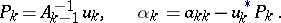The calculating scheme of the bordering method for the solution of a system is as follows. Let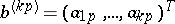,;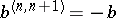. Ifis a non-degenerate matrix and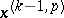is the solution of the system, then the solutionof the systemis obtained from the representation(3)

and from (2) as follows(4)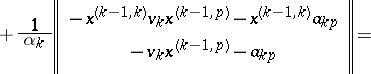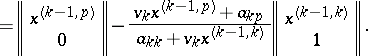In this way, through the solutionsand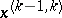of the systems with the same matrixand with various right-hand sides, it is easy to obtain the solution of the system with the bordered matrix. The solution of the initial system is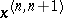. This can be obtained by the recurrent use of relation (4). This leads to the subsequent calculation of the set of vectors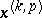,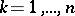,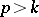, that isIn the amount of calculation necessary, this bordering method is equivalent to the Gauss method, one of the fastest direct methods of solving systems.

The bordering method allows one to solve higher-order systems owing to the effective use of computer memory. This is caused by the fact that for the calculation of the vectors,, only the storage of the vectors,, and the coefficients of a-th order system of equations is necessary, that is, a series of numbers of length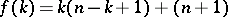. It is therefore sufficient to have a working capacityto solve a system of order. Moreover, the elements of the matrix and of the right-hand side do not have to be put into the computer memory immediately but successively, row by row.

It is advisable to use the bordering method when solving systems for which a truncated system has already been solved. Relation (4) then immediately gives the required solution.

The scheme of the bordering method described can be used to calculate the determinant. It follows from (1) that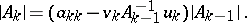Recurrent use of this relation gives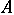.

As with the inversion of a matrix, the solution of a system and the calculation of the determinant by means of the bordering method is possible only for matrices with non-zero principal minors. It is generally also necessary here to use a scheme with a choice of a principal element.